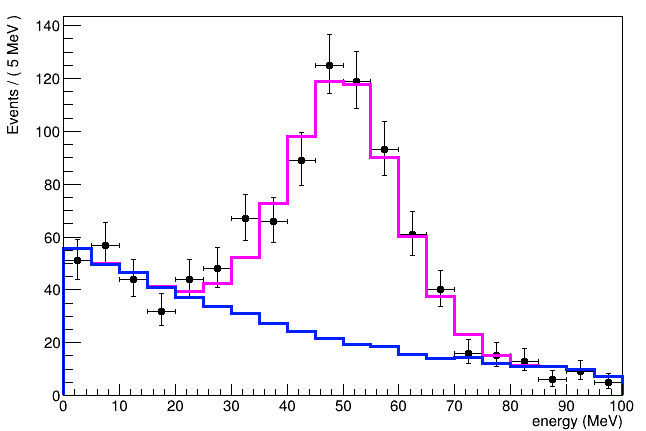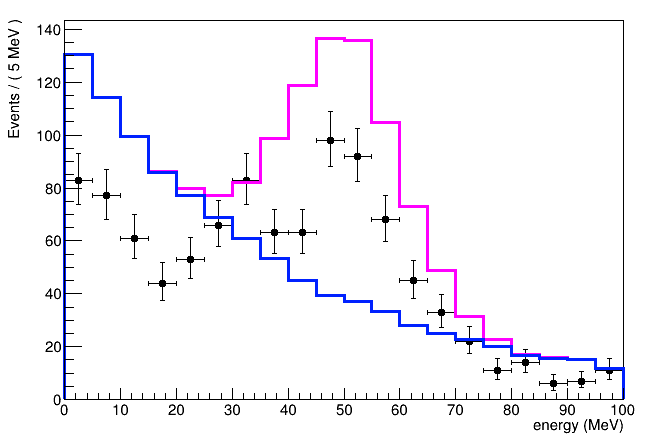# RooFit: include shape systematics

Dear all,

I am trying to create a model like this (f*signal + (1-f)*background). Other than the parameter of interest, the signal fraction, I need to include also a nuisance parameter, accounting for the systematics on the background shape.

The background shape variation is defined by a nominal distribution, and the two distributions corresponding to ±1 sigma variations, respectivelly. So I am using the PiecewiaeInterpolation class to model the shape systematic through a parameter alpha.

From this model, I want to generate data, which then I want to use to make fit studies.

The problem I have is that when I set a value of alpha different than zero to generate the data, I get weird behaviour: data and model do not match. What am I missing?

Here is a sample code:

``````void test_morph()
{
// signal
TH1F* hsgn = new TH1F("hsgn","signal", 20, 0, 100);
TF1* g = new TF1("g","*exp(-(x-)^2/(2*^2))", 0, 100);
g -> SetParameters(100, 50, 10);
hsgn -> FillRandom("g", 10000);

// background
TH1F* hbkg_nom = new TH1F("hbkg_nom","nominal bkg", 20, 0, 100);
TF1* fe = new TF1("fe","*exp(-*x)", 0, 100);
fe -> SetParameters(50, 0.02);
hbkg_nom -> FillRandom("fe", 10000);

TH1F* hbkg_p = new TH1F("hbkg_p","+1 sigma bkg", 20, 0, 100);;
fe -> SetParameters(55, 0.03);
hbkg_p -> FillRandom("fe", 10000);

TH1F* hbkg_m = new TH1F("hbkg_m","-1 sigma bkg", 20, 0, 100);;
fe -> SetParameters(45, 0.01);
hbkg_m -> FillRandom("fe", 10000);

// define observables
RooRealVar e{"e", "energy", 0, 100, "MeV"};
e.setBins(Int_t(20));

// signal pdf
RooDataHist dh_sgn{"dh_sgn", "dh_sgn", RooArgSet{e}, hsgn};
RooHistPdf sgn("sgn", "sgn", RooArgSet{e}, dh_sgn);

// background pdf
//
// nominal value
RooDataHist dh_bkg_nom{"dh_bkg_nom", "dh_bkg_nom", RooArgSet{e}, hbkg_nom};
RooHistPdf bkg_0("bkg_0", "bkg_0", RooArgSet{e}, dh_bkg_nom);
//
// bkg +1 sigma
RooDataHist dh_bkg_p{"dh_bkg_p", "dh_bkg_p", RooArgSet{e}, hbkg_p};
RooHistPdf bkg_p("bkg_p", "bkg_p", RooArgSet{e}, dh_bkg_p);
//
// bkg -1 sigma
RooDataHist dh_bkg_m{"dh_bkg_m", "dh_bkg_m", RooArgSet{e}, hbkg_m};
RooHistPdf bkg_m("bkg_m", "bkg_m", RooArgSet{e}, dh_bkg_m);
//
// build interpolation between -1 -> +1 sigma
RooRealVar alpha{"alpha", "alpha bkg sys", 0, -5, 5};
PiecewiseInterpolation bkg_sys("bkg_sys", "bkg_sys", bkg_0, bkg_m, bkg_p, alpha);

// build model
RooRealVar f{"f", "f", 0.5, 0, 1};
RooAddPdf model{"model", "model", RooArgSet{sgn, bkg_sys}, f};

alpha.setVal(0.0);
RooDataHist* data = model.generateBinned(RooArgSet{e}, 1000, RooFit::Verbose(1));

TCanvas* c = new TCanvas();
c->cd();

auto frame = e.frame();

data->plotOn(frame);

model.plotOn(frame, RooFit::LineColor(6));
model.plotOn(frame, RooFit::LineColor(4), RooFit::Components("bkg_sys"));

frame -> Draw();
}
``````

These is what I get with alpha = 0:and this the output with alpha > 0:The PiecewiseInterpolation is not a PDF that you can generate events from (or fit to, for that matter). In my version of ROOT, I immediately get

``````[#0] ERROR:InputArguments -- RooAddPdf::RooAddPdf(model) last argument bkg_sys is not of type RooAbsPdf.
terminating with uncaught exception of type std::invalid_argument: Last argument for RooAddPdf is not a PDF.
``````

This check was probably added later, which is why you don’t see it.

The solution is to use a RooRealSumPdf check its docs for an explanation why you need it.

See here what I changed to get the plot below.

``````-void test_morph()
+void testData()
{
// signal
TH1F* hsgn = new TH1F("hsgn","signal", 20, 0, 100);
@@ -48,10 +48,12 @@

// build model
RooRealVar f{"f", "f", 0.5, 0, 1};
-RooAddPdf model{"model", "model", RooArgSet{sgn, bkg_sys}, f};
+	RooRealSumPdf model{"model", "model", RooArgSet{sgn, bkg_sys}, f};

alpha.setVal(0.0);
RooDataHist* data = model.generateBinned(RooArgSet{e}, 1000, RooFit::Verbose(1));
+	alpha.setVal(1.0);
+	RooDataHist* data2 = model.generateBinned(RooArgSet{e}, 1000, RooFit::Verbose(1));

TCanvas* c = new TCanvas();
c->cd();
@@ -59,6 +61,7 @@
auto frame = e.frame();

data->plotOn(frame);
+  data2->plotOn(frame, RooFit::LineColor(kRed), RooFit::MarkerColor(kRed));

model.plotOn(frame, RooFit::LineColor(6));
model.plotOn(frame, RooFit::LineColor(4), RooFit::Components("bkg_sys"));
``````

Hi @StephanH,

thanks for the explanation.

In the next step I am trying to introduce a gaussian constraint on alpha, and performing a fit on the generated data. I am following this tutorial:

https://root.cern/doc/master/rf604__constraints_8C.html

This is the code, merged with your changes:

``````void test_morph()
{
// signal
TH1F* hsgn = new TH1F("hsgn","nominal bkg", 20, 0, 100);
TF1* g = new TF1("g","*exp(-(x-)^2/(2*^2))", 0, 100);
g -> SetParameters(100, 50, 10);
hsgn -> FillRandom("g", 10000);

// background
TH1F* hbkg_nom = new TH1F("hbkg_nom","nominal bkg", 20, 0, 100);
TF1* fe = new TF1("fe","*exp(-*x)", 0, 100);
fe -> SetParameters(50, 0.02);
hbkg_nom -> FillRandom("fe", 10000);

TH1F* hbkg_p = new TH1F("hbkg_p","+1 sigma bkg", 20, 0, 100);;
fe -> SetParameters(55, 0.03);
hbkg_p -> FillRandom("fe", 10000);

TH1F* hbkg_m = new TH1F("hbkg_m","-1 sigma bkg", 20, 0, 100);;
fe -> SetParameters(45, 0.01);
hbkg_m -> FillRandom("fe", 10000);

// define observables
RooRealVar e{"e", "energy", 0, 100, "MeV"};
e.setBins(Int_t(20));

// signal pdf
RooDataHist dh_sgn{"dh_sgn", "dh_sgn", RooArgSet{e}, hsgn};
RooHistPdf sgn("sgn", "sgn", RooArgSet{e}, dh_sgn);

// background pdf
//
// nominal value
RooDataHist dh_bkg_nom{"dh_bkg_nom", "dh_bkg_nom", RooArgSet{e}, hbkg_nom};
RooHistPdf bkg_0("bkg_0", "bkg_0", RooArgSet{e}, dh_bkg_nom);
//
// bkg +1 sigma
RooDataHist dh_bkg_p{"dh_bkg_p", "dh_bkg_p", RooArgSet{e}, hbkg_p};
RooHistPdf bkg_p("bkg_p", "bkg_p", RooArgSet{e}, dh_bkg_p);
//
// bkg -1 sigma
RooDataHist dh_bkg_m{"dh_bkg_m", "dh_bkg_m", RooArgSet{e}, hbkg_m};
RooHistPdf bkg_m("bkg_m", "bkg_m", RooArgSet{e}, dh_bkg_m);
//
// build interpolation between -1 -> +1 sigma
RooRealVar alpha{"alpha", "alpha bkg sys", 0, -5, 5};
PiecewiseInterpolation bkg_sys("bkg_sys", "bkg_sys", bkg_0, bkg_m, bkg_p, alpha);

// build model
double f_val = 0.5;
RooRealVar f{"f", "f", f_val, 0, 1};
RooRealSumPdf sb{"sb", "sb", RooArgSet{sgn, bkg_sys}, f};

// introduce alpha constraint
RooGaussian gaus_alpha("gaus_alpha", "gaus_alpha", alpha, RooFit::RooConst(0), RooFit::RooConst(1));
RooProdPdf model{"model", "model", sb, gaus_alpha};

alpha.setVal(1);
RooDataHist* data = model.generateBinned(RooArgSet{e}, 1000, RooFit::Verbose(1));

TCanvas* c = new TCanvas();
c->cd();

auto frame = e.frame();

data->plotOn(frame);

model.plotOn(frame, RooFit::LineColor(6));
model.plotOn(frame, RooFit::LineColor(4), RooFit::Components("bkg_sys"));

frame -> Draw();

// fit
f.setVal(f_val+0.05);
alpha.setVal(0.9);
//alpha.setConstant(kTRUE);

auto fitres = model.fitTo(*data, RooFit::Constrain(alpha), RooFit::Save());

fitres->Print();

TCanvas* c1 = new TCanvas();
c1->cd();

auto frame1 = e.frame();

data->plotOn(frame1);

model.plotOn(frame1, RooFit::LineColor(6));
model.plotOn(frame1, RooFit::LineColor(4), RooFit::Components("bkg_sys"));

frame1 -> Draw();

}
``````

The fit does not converge, but I am not able to track the problem: I see different warning during the fit like:

``````[#0] WARNING:Minization -- RooMinimizerFcn: Minimized function has error status.
``````

but can’t figure out what is going on.

Thanks!

Usually, when you get evaluation errors, there is more info before as to what’s going wrong. Check the logs for warnings/errors.
A frequent problem is a PDF that becomes negative (that’s not a PDF, any more). Check if you have components whose sum is below zero.

ok, thanks @StephanH.

If I use RooHistFunc in place of RooHistPdf for the components of the model, then the fit converges.

I attach the log files for both cases.

test_histpdf.txt (36.1 KB)
test_histfunc.txt (5.5 KB)

Yes, similar to the difference between RooAddPdf and RooRealSumPdf, RooHistPdf is not allowed to be negative whereas RooHistFunc is. As long as the sum of all the HistFuncs is positive, that’s fine.

This topic was automatically closed 14 days after the last reply. New replies are no longer allowed.